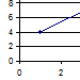Performance Engineering and Toilets

I was reading the paper on Saturday and noticed this snippet about student Li Tingting occupying a men’s public toilet to protest about unequal waiting times. Local Officials have promised to increase the number of Ladies toilets by 50% to decrease waiting times. Strange to some but some of the calculations we use in performance engineering can help calculate if 50% is enough to reduce waiting times.Using queuing theory a branch of mathematics, that allows us to calculate the waiting times if we know the arrival rate and the time customers spend being “serviced.” There are other consideration when using these calcuations so google “queueing theory”.

We know the “service time” for ladies and gentlemen using toilets thanks to studies done in New Zealand where it is a legal requirement that there should be a sufficient number of public toilet that the average time taken by a man is 40 seconds while it takes 90 seconds by a woman. The calculation below can be used for a single toilet and gives the time in the system for a know arrival rate and service rate.For a male toilet we can service 90 men per hour (3600 seconds in an hour divided by 40 seconds per visit) and for a female toilet we can service 40 women per hour (3600 seconds divided by 90). For a range of arrival rates using the calculation above we can calculate the average time in the system (waiting plus doing business) and plot this on the graph below:As you can see as arrival rate increases the time women spend increases more quickly. Also note how it gets worse as the arrival rate approaches the service rate.

The example above is just for a single toilet example, but there are equations that can calculate the time for multiple service centers (in this case toilets) and therefore can be used to calculate if a 50% increase in women’s toilets would reduce waiting times. Well the devil is in the details as to whether 50% is sufficient based on current arrival rates, current number of male/female toilets but I thought let’s try a few numbers. Let’s assume there is currently 10 toilets per sex and therefore we need to look at the time spent for a range of arrival rates for 10 male toilets and 15 female toilets. The graph below shows the times for different arrival rates.As you can see the time significantly increase for ladies well before that of the men! 50% May NOT be enough.

OK, so why is an IT performance engineering blog talking about Chinese toilets. Well it is just an example of how some equations can be used to calculate things like response times where there are limited resources. Just like when considering “how many CPUs” you need for applications the same math can used.